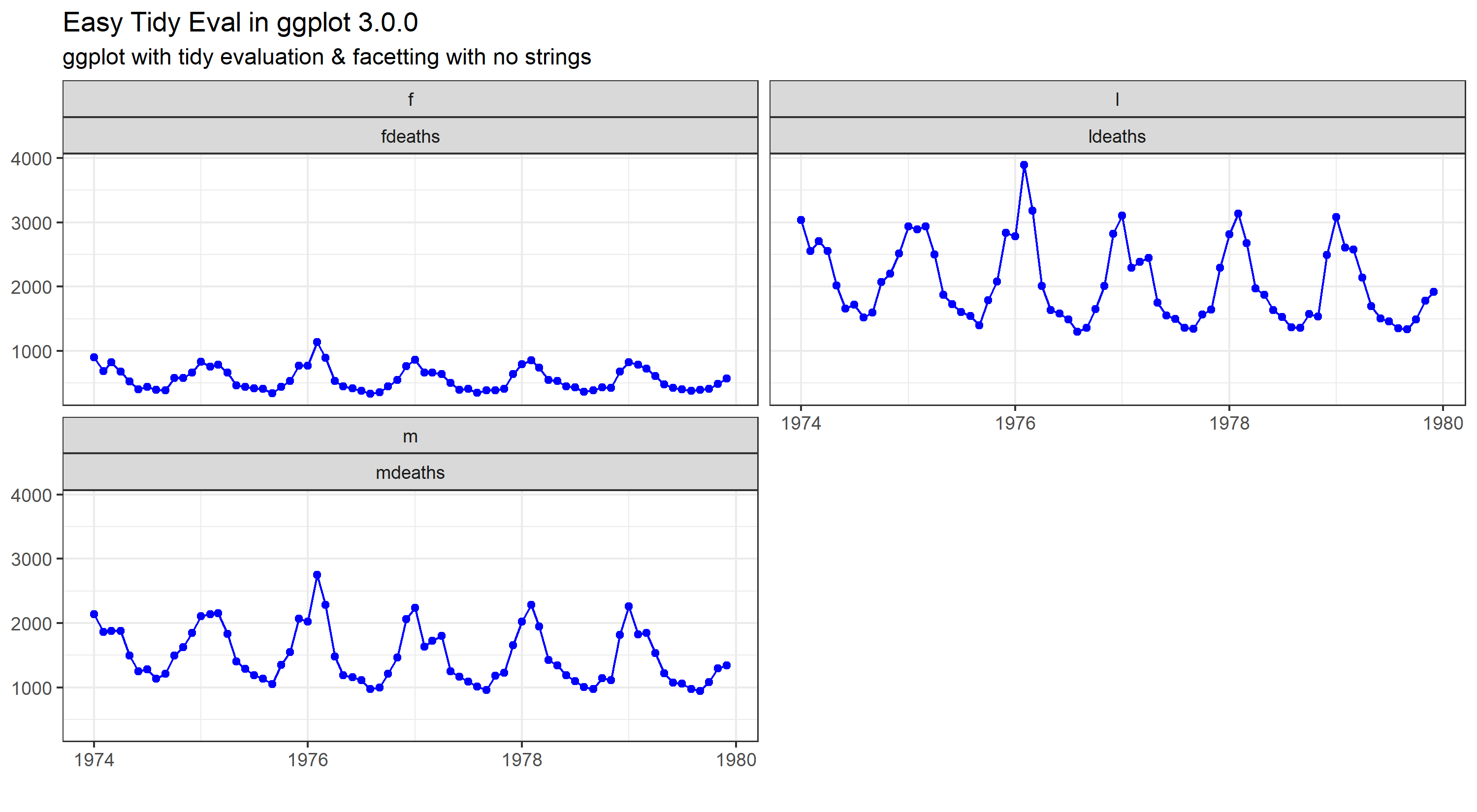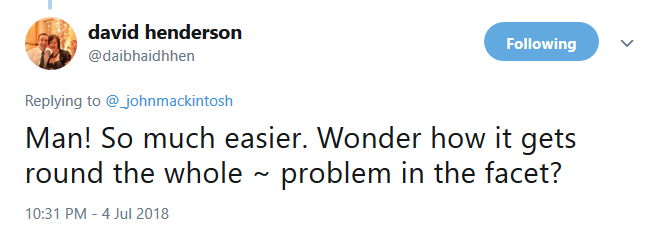# More tidy evaluation with ggplot2Another look at tidy evaluation with a slight twist on the previous post

After my previous post, my good friend David Henderson asked a good question on Twitter ( it happens :))To be honest, I hadn’t even noticed there was no tilde (the ‘~’ ) in my call to facet_wrap.

So I did a little experiment :

library(dplyr)
library(ggplot2)
library(tidyr)
library(tibble)

data <- list(fdeaths, mdeaths, ldeaths) #time series data - needs prep

names(data)[1:3] <- c("fdeaths", "mdeaths", "ldeaths")

data <- as_tibble(data)
startdate <- as.Date('1974-1-1')
data$date <- seq.Date(startdate,by = 'month',length.out = 72) newdata <- tidyr::gather(data, key = key, value = value,-date) newdata$value <- as.numeric(newdata$value) # adding in an additional colum to facet by newdata$random <- stringr::str_sub(newdata\$key,1,1)

gtest <- function(df,x,y,...) {
# check out the ... in the function parameters
x_quo <- enquo(x)
y_quo <- enquo(y)
groupvars <- quos(...) ## this is a new bit too

p <- ggplot(df,aes(x = !!x_quo, y = !!y_quo)) +   #bangin'
geom_line(colour = "blue", group = 1) +
geom_point(colour = "blue") +
facet_wrap(groupvars, ncol = 2) #look Ma, no tilde. Also, 2 cols, not 3

p <- p + ggtitle(label = "Easy Tidy Eval in ggplot 3.0.0",
subtitle = "ggplot with tidy evaluation & facetting with no strings")

p <- p + labs(x = NULL, y = NULL, caption = "") +
theme_bw()

print(p)
}

gtest(newdata, date, value, random, key)Pretty cool huh?

What’s different here?

Well, I added in ‘…’ to the definition, and assigned groupvars using quos(…) Prior to that I’d taken the first letter of the key column as a new additional grouping column. So now we have 2 columns to facet_wrap.

Normally we’d use

facet_wrap(random ~ key)


Or vice versa. But, I didn’t need to do that, I just specified ‘groupvars’ as the faceting variable, and changed the number of columns to prove it actually does what I want.

facet_wrap(groupvars, ncol = 2)


Cleverer people than me will be able to explain this magic, but in the meantime, if you need to create a faceted ggplot2 function, I hope this post and the previous one help you get off the ground.

If you like this, please consider liking it (wherever is appropriate) and / or sharing it. Tag me on Twitter if you do ! @_johnmackintosh

Also clever peeps are welcome to explain the magic in the comments down below.

Cheers :)